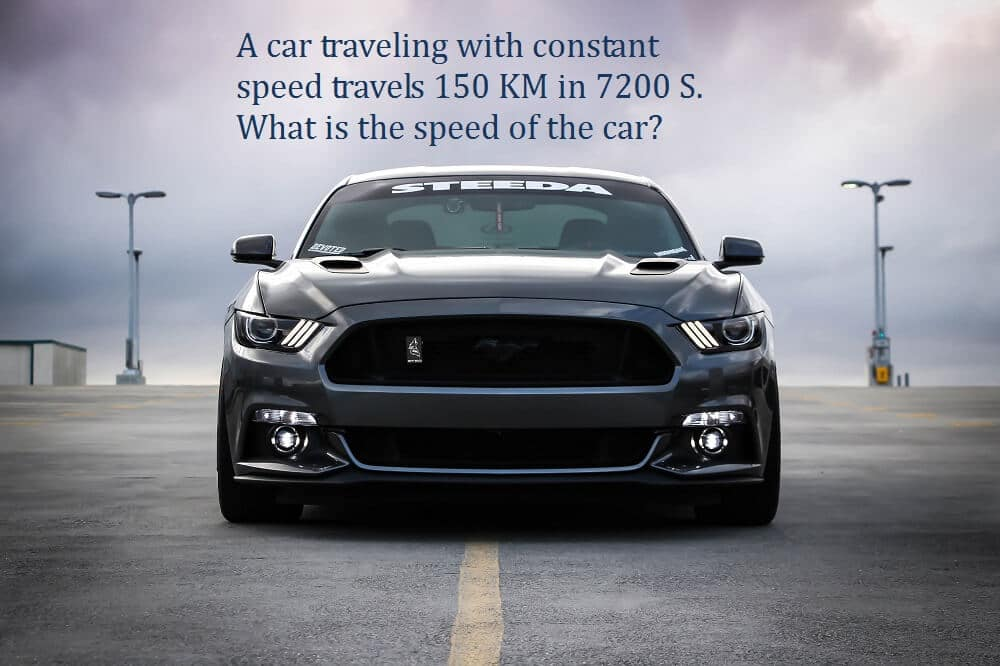Sat. Oct 23rd, 2021

# A car traveling with constant speed travels 150 KM in 7200 S. What is the speed of the car?Let’s solve the following sum:

A car traveling with constant speed travels 150 KM in 7200 S. What is the speed of the car?

Data:

Distance travelled = 150 KM = 150,000 M

Time taken         = 7200 S

To Find:

Speed of the Car=?

Solution:

A car traveling with constant speed travels 150 KM in 7200 S. What is the speed of the car?  here is the formula that is used to find the speed of the car

Speed of the Car =   Distance travelled/ time taken

Now, put the values of different variables and then process the calculations in order to find the solution of A car traveling with constant speed travels 150 KM in 7200 S. What is the speed of the car?

Speed of the car = 150,000/7200

20.833 m/s

The above simple solutions have given the answer of: A car traveling with constant speed travels 150 KM in 7200 S. What is the speed of the car?

Speed of the car= 20.833 m/s

In other words, the car is moving with a speed of 20.833 meter per second.

I hope you have find the solution of: A car traveling with constant speed travels 150 KM in 7200 S. What is the speed of the car?

## Alternative Solution of the question:A car traveling with constant speed travels 150 KM in 7200 S. What is the speed of the car?

If you want to find the speed of the car in KM/Hours, then use the following method:

Data:

Distance Covered= 150 KM

Time taken= 7200 S

Solution:

Change the time in seconds to the time in hours.

For this, use the following formula:

Time in hours = Time in seconds/ seconds in one hour

Time in hours= 7200/3600

Time in hours= 2 hours.

Now find the speed as follows:
Speed of the car = Distance travelled/time taken

Speed of the car= 150 KM/2 hours

Speed of the Car= 75 KM/Hour

So, we found the answer of: A car traveling with constant speed travels 150 KM in 7200 S. What is the speed of the car?  And the car is moving with a speed of 72 KM/ Hours or at the rate of 20.833 m/s